Courses

# Differential Equations - 8

## 20 Questions MCQ Test Topic-wise Tests & Solved Examples for IIT JAM Mathematics | Differential Equations - 8

Description
This mock test of Differential Equations - 8 for Mathematics helps you for every Mathematics entrance exam. This contains 20 Multiple Choice Questions for Mathematics Differential Equations - 8 (mcq) to study with solutions a complete question bank. The solved questions answers in this Differential Equations - 8 quiz give you a good mix of easy questions and tough questions. Mathematics students definitely take this Differential Equations - 8 exercise for a better result in the exam. You can find other Differential Equations - 8 extra questions, long questions & short questions for Mathematics on EduRev as well by searching above.
QUESTION: 1

Solution:
QUESTION: 2

Solution:
QUESTION: 3

### The equation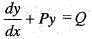is linear differential equation of first order, if

Solution:
QUESTION: 4

An integrating factor of x dy/dx + (3x + 1)y = xe–2x is

Solution: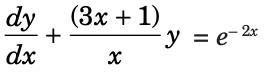which is a linear differential equation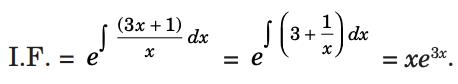QUESTION: 5

What is the reason behind the non-existence of any real function which satisfies the differential equation, (y’)2 + 1 = 0?

Solution:

Given: (y’)2 + 1 = 0 Consider if y = 2x, then y’ = 2 and hence the left-hand side of the equation becomes 3 which is greater than 1. Therefore, the left-hand side of the equation will always be greater than, or equal to one and thus cannot be zero and hence the differential equation is not satisfied.

QUESTION: 6

What is the order of the partial differential equation,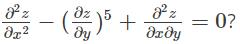Solution:

The order of an equation is defined as the highest derivative present in the equation. Hence, in the given equation,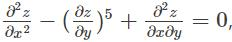the order is 2.

QUESTION: 7

A particular integral of y" - (a + b)y' + aby = Q(x) is,

Solution:
QUESTION: 8

The homogeneous differential equation M(x, y)dx, N(x, y) dy = 0 can be reduced to a differential equation, In which the variable are separated, by the substitution

Solution:
QUESTION: 9

General solution of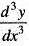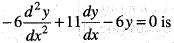Solution:
QUESTION: 10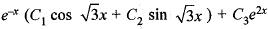is the general solution of

Solution:
QUESTION: 11

In linear ordinary differential equation, the dependent variable and its differential coefficients are not multiplied together and occurs only in

Solution:
QUESTION: 12

General solution of the equation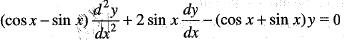Given that y = sin x is a solution, is

Solution:
QUESTION: 13

Solve d3y/dx3 - d2y/dx2 - 4dy/dx + 4y = 0 has the solution

Solution:

Given equation is (D3 – D2 – 4D + 4)y = 0

A.E. is m3 – m2 – 4m + 4 = 0  m2(m – 1) – 4(m – 1) = 0

(m – 1) (m2 – 4) = 0

m = 1, m = ± 2

m1 = 1, m2 = 2, m3 = – 2

∴ The general solution of the given equation is y = C1ex + C2 e2x + C3 e-2x

QUESTION: 14

The P.I. of the differential equation (D3 - D)y = ex + e-x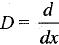is

Solution:
QUESTION: 15

If φ(x, y) = 0 is a singular solution, then φ(x, y) is a factor of

Solution:
QUESTION: 16

P.I . of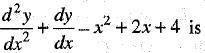Solution:
QUESTION: 17

The complementaiy function of (D4 - a4)y = 0 is

Solution:
QUESTION: 18

A differential equation of first order and first degree is homogeneous, if

Solution:
QUESTION: 19

The integrating factor for the differential equation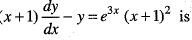Solution:
QUESTION: 20

Given, an equation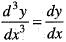and a solution of it is y = a0 + a1 sinh x + a2 cosh x, where a0, a1, a2 are arbitrary constants, then this solution is

Solution: